# Theoretical investigation of a ceramic monolith heat exchanger using silicon carbide and aluminium nitride as heat exchanger material

Theoretical investigation of a ceramic monolith heat exchanger using silicon carbide and aluminium nitride as heat exchanger material

Umayorupagam P. Arunachalam Mohan Edwin*

Department of Mechanical Engineering, University College of Engineering, Nagercoil 629 004, India

Anna University Constituent College, Konam, Nagercoil 629 004, India

Corresponding Author Email:
edwinme1980@gmail.com
Page:
645-650
|
DOI:
https://doi.org/10.18280/ijht.350323
|
Accepted:
|
Published:
30 September 2017
| Citation

OPEN ACCESS

Abstract:

Ceramic heat exchangers are preferred in most high temperature applications due to its high temperature stability and corrosion resistance. This work investigated the performance of a ceramic heat exchanger, evaluating the heat transfer and pressure drop theoretically by Ɛ-NTU method by using silicon carbide and aluminium nitride as heat exchanger material. The performances of both the heat exchangers are correlated. The heat exchanger of cross flow type considered for this study entails of rectangular ducts for the passage of exhaust gas, a ceramic core, and the air passage through rectangular ducts. The heat exchangers were studied using conventional Ɛ-NTU method with numerous Nusselt analogies to characterize the flow in the rectangular duct. The theoretical analyses reveal that, the performance parameters such as overall heat transfer increased by 4.5-5%, effectiveness by 3% and heat transfer rate by 3% using aluminium nitride as the heat exchanger material relative to silicon carbide.

Keywords:

ceramic recuperator, cross flow heat exchanger, effectiveness, heat transfer, pressure drop

1. Introduction

The energy consumption of the world is rapidly increasing due to the population and the increase in industries. The fossil fuels are normally utilized to generate the power. Due to the high consumption of the fossil fuels by the power sectors, this will be exhausted in future lead to global warming and environmental pollution. Hence the investigation towards fuel systems attains important in the energy sector as an alternative energy sources. Solid oxide fuel cells (SOFC) are more useful as it has 60% of electric conversion efficiency. This SOFC requires recuperators to recover the high heat energy available from the exhaust gases having the temperature range of 600-1000°C.

Accordingly, high thermal resistance materials are essential to construct the heat exchangers subjected to high heat flux. The SOFC/GT hybrid power generating systems including gas turbines is used to generate electricity from the recovered heat.

Nowadays, a hybrid recuperators are very much essential in the power generation systems. Hybrid recuperators has three pass systems , first is through the ceramic heat exchanger with a temperature between 600°C and 1000°C and the other two flows with metallic heat exchangers having a working temperature range of 150°C and 600°C and below 150°C.

Numerous investigations have been carried out to assess the performance of recuperators. Most of them are focused towards the efficiency improvement of the systems. The heat flux and the flow turbulence of the gas having various cross-sectional areas are simulated by Rokni and Sunden . They also used different turbulence model implementing the significant use of thermal boundary conditions. The combined effects of longitudinal heat conduction, inlet flow deviation from ideality and temperature gradient along the flow direction on the performance of the cross-flow plate heat exchanger was investigated by Ranganayakulu et al . Hetsroni et al.  carried out different experiments to analyze the flow variation due to the pressure fluctuations. Rashidi et al  carried out the sensitivity analysis using Response Surface Methodology (RSM). In this work, Reynolds and Darcy numbers and porous substrate thicknesses are selected as the influence parameters. Bourisa et al.  optimized the tube shape from the three different cross sections considered by achieving higher heat flux. Park et al.  performed different analysis with a simple rectangular finned-type heat exchanger model with aid of CFD simulation and achieved highly efficient ceramic heat exchanger for their research. Jin GiPaeng et al.  havestated that heat resistant material is necessary for the construction of high temperature heat exchangers and assessed the performance of a ceramic monolith heat exchanger, estimating heat transfer and pressure drop by numerical computation and the ε-NTU method. Hamed Sadight Dizaji at al  shows that the air bubble injection and bubbles mobility (because of buoyancy force) can intensify the NTU and exergy loss by mixing the thermal boundary layer and increasing the turbulence level of the fluid flow. VijaisriNagarajan et al.  have predicted that the thermal–hydraulic performances of compact surface heat exchangers are strongly influenced by their geometry and flow configurations.WenJian et al  showed that the shell-side tangential velocity and radial velocity in improved heat exchanger increase significantly and the shell-side fluid becomes approximately continuous spiral flow.Hameed B.Mahood et al  investigated the heat transfer efficiency, and hence cost, of a three-phase direct contact condenser has been carried out utilising a low grade energy sources. Paulo Eduardo Batista et al.  havestated ceramic materials are the actual natural choice for the high temperature heat exchanger (HTHE). This work presented one thermodynamic study of one EFGT (Externally Fired Gas Turbine) with one detailed model for the ceramic heat exchanger.ZengliGao et al.  have studied the influence of honeycomb ceramic on heat extraction numerically on the basis of experimental verification of mathematical model.Ting Ma et al. have studiedthe effects of inlet temperature and rib height on the fluid flow and heat transfer performances of the ribbed channel inside the high temperature heat exchanger. Yonatan Cadavid et al.  have fabricated honeycomb matrix from alumina for waste heat recovery system and developed a model to analyse the performance of the heat exchanger and design a calculation algorithm for the compact heat recovery units. Thomas Fend et al.  have developed the idea of creating an advanced geometry for a heat exchanger by taking the extruded 2D honeycomb structure as a basis from a receiver system which aims at transferring heat from concentrated radiation to an air circuit, which feeds the boiler of a steam turbine.Robert J. Kee et al.  have designed, fabricated and evaluated a ceramic counter-flow microchannel heat exchanger; the work reports the model-based design and experimental performance evaluation of an all-ceramic compact counter-flow micro-channel heat exchanger. Yanpeng Ji et al.  performed analysis with heat exchangers having fined tube arrangements by using novel bayonet tube for high heat load conditions which can be used high temperature atmosphere, reactors and for externally fired combined cycle. Y. Takeuchi et al.  have investigated numerically the performance of a compact SiC heat exchanger for a wide range of thermal media, liquid LiPb and helium gas, flow rates for its development. The potential of porous structures (e.g., metallic foam) in heat exchangers is currently undergoing growing interest due to their large surface area per unit of volume. Recently tremendous works have been conducted on heat transfer enhancement and a large number of techniques for heat transfer enhancement have been developed .

Most of the research papers computed the heat transfer characteristics and performance analysis of the heat exchangers. However, in this paper, we conducted computational numerical analysis of the heat transfer for a monolith and AlN heat exchanger. Furthermore, the heat transfer rate was also evaluated using ε-NTU method, using various Nusselt number correlations, compared with numerical computations.

In this paper, performance analysis of a monolithic heat exchanger with rectangular channels using the ε-NTU theoretical method is presented. The different Nusselt analogies are used to investigate the performance. Ceramic heat exchangers have low thermal efficiency than the metallic heat exchangers but benefits of cheap material cost. Apart from the heat transfer performance, the pressure drops of the ceramic heat exchanger including three pass recuperators was also analyzed. The overall heat transfer coefficient “U” is assumed to be constant for all the theoretical calculations during the flow of working fluid through the heat exchanger. The different Nusselt analogies and various boundary conditions may lead to uncertainty (may lead to even 30 percent variation in the predicted value of overall heat transfer coefficient). Hence it is essential to overdesign the heat exchangers systems to avoid unpleasant surprises. The considerations are also focused in pressure drop and pumping power. Therefore, any gain from the enhancement in heat transfer should be weighed against the pumping cost resulting due to the pressure drop. The selection of heat exchanger for a particular situation is a huge task for design engineers as they have to balance heat transfer task with proper matching of components in the system.

2. Theoretical Analysis

The ceramic recuperator consists of rectangular hot stream exhaust and cold air passages with the exhaust and air in cross flow direction without mixing each other as shown in Figure 1.

## 1.pngFigure 1. Schematic diagram of the ceramic heat exchanger

2.1 Overall heat transfer coefficient (U)

The overall heat transfer coefficient, U, between hot and cold fluids is a principal factor in estimating the rate of heat transfer. It is expressed as Eq. (1)

$\mathrm{U}=\frac{1}{\frac{1}{\mathrm{hair}}+\frac{\Delta \mathrm{t}}{\mathrm{k}}+\frac{\mathrm{A}_{\mathrm{air}}}{\eta_{\mathrm{t}} \mathrm{A}_{\mathrm{gas}} \mathrm{h}_{\mathrm{gas}}}}$       (1)

where k is the thermal conductivity of the ceramic core, Ƞt is the total surface effectiveness of a fin, △t is the thickness of the wall, A air & A gas are the air-side and the exhaust-side heat transfer areas, h air and h gas are each side average convective heat transfer coefficients, obtained from Nusselt relations of Eq. (2).

$\mathrm{h}=\mathrm{Nu} \times \frac{\mathrm{k}}{\mathrm{D}_{\mathrm{h}}}$    (2)

where k is the thermal conductivity of each fluid, Dh is a hydraulic diameter of the rectangular fluid passage. Correlations equations of the Nusselt number from literature are listed in Table 1. The equations in Table 1 were derived under fully developed or developing flow conditions with constant wall heat transfer rate (q”=const).

Table 1. Correlations of Nusselt numbers in duct as reported in the literature [7, 8]

 Reference Correlation Conditions Range of validity Geometry Flow regime Kays and Crawford Nu=8.325(1-1.883/α +3.767/α2 -5.814/α3 +5.361/α4-2/α5) Rectangular Fully developed (constant wall heat flux) Re<2200 Sieder-Tate correlation $N u=1.86\left(R e P r D_{\mathrm{h}} / L\right)^{0.33}\left(\mu_{\mathrm{f}} / \mu_{\mathrm{w}}\right)^{0.14}$ Circular Simultaneously developing    Constant wall temperature Re<2200 Stephan correlation $\mathrm{Nu}=4.364+\frac{0.086\left(\mathrm{RePrD}_{\mathrm{h} / \mathrm{L}}\right)^{1.33}}{1+0.1 \mathrm{Pr}\left(\mathrm{ReD}_{\mathrm{h}} / \mathrm{L}\right)^{0.83}}$ Circular Simultaneously developing    Constant wall temperature 0.77) Shah and London $N u=\left\{\begin{array}{c}{1.953\left(\operatorname{RePr} D_{\mathrm{h}} / L\right)^{0.33}} \\ {4.364+0.0722\left(\operatorname{RePr} D_{\mathrm{h}} / L\right)}\end{array}\right.$ Circular Thermally developing laminar      Constant wall temperature RePrD/L<33

2.2 Ɛ-NTU method

The actual heat transfer rate of the ceramic heat exchanger was determined from the theoretical relation of the ε -NTU method in which the effectiveness (ε) is shown in Eq. (3) for an unmixed fluid flow condition.

$\varepsilon=1-\exp \left\{\frac{\mathrm{NTU}^{0.22}}{\mathrm{C}}\left[\exp \left(-\mathrm{CNTU}^{0.78}\right)-1\right]\right\}$     (3)

where C is the ratio of heat capacities (Cmin/Cmax).

NTU is the ratio of the total conductance (UA) to the minimum heat capacity (Cmin) where Cmin is the lower heat capacity (m.Cp)min and Cmax is the higher heat capacity (m.Cp )max of the two fluids where m. and Cp are the mass flow rate and specific heat of the hot and cold fluids, respectively.

The actual heat exchange rate from hot to cold fluids can be obtained from Eq. (4).

$\mathrm{q}=\varepsilon \times \mathrm{C}_{\min }\left(\mathrm{T}_{\mathrm{gas}_{-} \mathrm{in}}-\mathrm{T}_{\mathrm{air}_{-}\mathrm{in}}\right)$   (4)

The exit terminal temperatures of exhaust and air (Tair-out and Tgas-out) are obtained from the inlet terminal temperatures of the fluids as Eq. (5) and Eq. (6).

$\mathrm{T}_{\mathrm{air}_{-} \mathrm{out}}=\mathrm{T}_{\mathrm{air}_{-} \mathrm{in}}+\frac{\mathrm{q}}{\mathrm{m}_{\mathrm{air}} \mathrm{C}_{\mathrm{p}} \mathrm{air}}$         (5)

$\mathrm{T}_{\mathrm{gas}_{-} \mathrm{out}}=\mathrm{T}_{\mathrm{gas}_{-} \mathrm{in}}+\frac{\mathrm{q}}{\mathrm{m}_{\mathrm{gas}} \mathrm{C}_{\mathrm{p}} \mathrm{gas}}$       (6)

2.3 Pressure drop

The Pressure drop is an important consideration in the heat exchanger design. The pressure drop across the heat exchanger is derived from the Darcy friction equation for pressure drop is as follows Eq. (7).

$\Delta \mathrm{P}_{\text { core }}=\mathrm{f} \frac{1}{2} \rho_{\mathrm{m}} \mathrm{V}^{2} \mathrm{m}\left(\mathrm{L} / \mathrm{D}_{\mathrm{h}}\right)$      (7)

where $\mathrm{f}=\frac{64}{\mathrm{Re}}$ since the laminar flow is considered in this study.

2.4 Ceramic core analysis

Before the investigation of the performance, the thermodynamic properties of the heat exchanging fluids were

arrived in two ways, one is to obtain the property values at average temperature of both inlet and outlet and the other is a linear function to the fluid temperature.

Table 2. Mass flow rate and corresponding Reynolds number

 Sl.No. Air stream Exhaust stream Re.No mair (kg/s) Re.No mgas (kg/s) 1 485 0.003966 2 606 0.004958 3 728 0.005950 79 0.003966 4 849 0.006942 5 970 0.007932

Table 3. Based on Kays and Crawford Nusselt number correlation

 Sl.No. Re.No U (W/m2 K) Ɛ- NTU Q (W) 1 485 23.86284 0.48 618.72 2 606 23.86284 0.50 657.24 3 728 23.86284 0.52 684.96 4 849 23.86284 0.54 705.47 5 970 23.86284 0.55 721.206

Table 4. Based on Sieder-Tate Nusselt number correlation

 Sl.No. Re.No U (W/m2 K) Ɛ- NTU Q (W) 1 485 15.073866 0.376 484.2 2 606 15.420896 0.394 515.17 3 728 15.985502 0.420 545.76 4 849 16.467688 0.435 570.08 5 970 16.887699 0.451 590.33

The theoretical analysis are performed for the exhaust mass flow rate of 0.003966 kg/s, with air flow rate from 0.003966 kg/s to 0.007932 kg/s in five steps. The Reynolds numbers concerned to the mass flow rates are presented in Table 4. All Reynolds numbers are based on average fluid temperatures. Reynolds number of 485 and a gas-side Reynolds number of 79, which indicates that the mass flow rates of the air and the exhaust, are the same at 0.003966 kg/s shown in the above Table 2.

The overall heat transfer coefficient, effectiveness and the heat transfer rate are determined using various Nusselt analogies. First the correlations are used for analyzing the heat exchanger using silicon carbide material and next for Aluminium nitride material. The theoretical observations are listed corresponding to the Reynolds number in the Tables3-6.

Table 5. Based on Stephan Nusselt number correlation

 Sl.No. Re.No U (W/m2 K) Ɛ- NTU Q (W) 1 485 19.944663 0.440 565.87 2 606 20.637231 0.465 609.614 3 728 21.272563 0.492 644.525 4 849 21.857679 0.514 673.011 5 970 22.397855 0.532 696.94

Table 6. Based on Shah and London Nusselt number correlation

 Sl.No. Re.No U (W/m2 K) Ɛ- NTU Q (W) 1 485 18.379366 0.420 541.814 2 606 18.851516 0.440 580.07 3 728 19.310297 0.466 610.64 4 849 19.756160 0.485 635.813 5 970 20.188913 0.501 657.249
3. Result and Discussion

The performance analysis of the heat exchanger was investigated for the exhaust flow rate of 0.003966 kg/s with varying the air flow rate from 0.003966 kg/s to 0.007932 kg/s in five steps by using different Nusselt analogies.

3.1 Analysis of the heat exchanger using SIC compared with ALN

First the heat exchanger is analysed using silicon carbide material and the performance of the heat exchanger using aluminium nitride was compared. The below graphical representations show the variation in overall heat transfer coefficient, effectiveness and heat transfer rate with respect to Reynolds number using various Nusselt number correlations.

The overall heat transfer coefficient obtained by using Kays and Crawford Nusselt number correlations was 23.863 W/m2K. For Sieder-Tate correlations the overall heat transfer coefficient varied from 15.07-16.89 W/m2K. Also for Stephan correlations it varied from 19.945-22.4 W/m2K and for Shah and London the overall heat transfer coefficient ranges from 18.38-20.19 W/m2K. Figure 2 shows the variation of overall heat transfer coefficient with Reynolds Number for the various correlations. In this figure, the total heat transfer coefficient calculated by Kays and Crawford is the highest and is invariant regardless of Reynolds number, Since the correlation is realized under fully developed flow conditions. All the other lines increasde towards the reynolds number, because they are under developing flow conditions.

The effectiveness obtained byKays and Crawford Nusselt number correlations varied from 0.48-0.55. For Sieder-Tate correlations the effectiveness varied from 0.38-0.45. Also for Stephan correlations it varied from 0.44-0.532 and by Shah and London the effectiveness ranges from 0.42-0.50 and the same is indicated from the Figure 3.

The Figure. 4 shows the heat transfer values obtained from different correlations with various Reynolds Number. The heat transfer rate by using Kays and Crawford Nusselt number correlations varied from 618.72-721.21 while a range of 484.2-590.33 W was observed bySieder-Tate correlations.It was noticed that 565.81-696.94 W of heat transfer by Stephan correlationand same was reduced [541.814-657.249 W] in Shah and London correlations.

## 2.png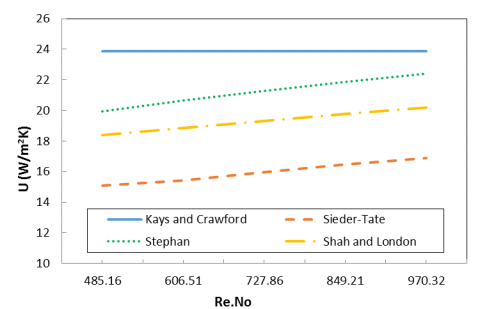Figure 2.Variation of overall heat transfer coefficient with Reynolds number

## 3.png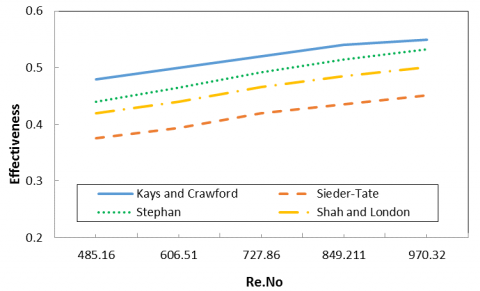Figure 3.Variation of effectiveness with Reynolds number

## 4.png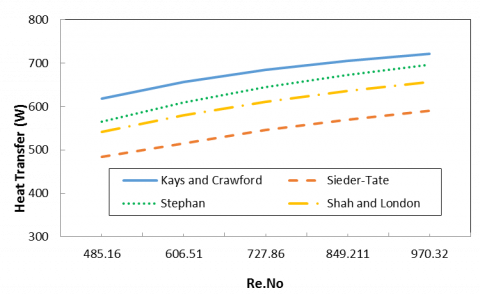Figure 4. Variation of heat transfer rate with Reynolds number

3.2. Performance comparison of the heat exchanger with SIC and ALN

Finally the performance parameters of the aluminium nitride heat exchanger were correlated with silicon nitride heat exchanger. The aluminium nitride heat exchanger showed enhanced performance such as improved overall heat transfer coefficient,effectiveness and heat transferof the system and was indicated clearly from the Figures 5 - 7.

The main reason for selecting aluminium nitride as heat exchanger material is due to its higher thermal conductivity, increased corrosion resistance and higher temperature withstanding capacity.

The overall heat transfer coefficient of the heat exchanger using aluminium nitride (AlN) material has increased by 4.5-5% when compared to Silicon carbide (SiC) material. The effectiveness of the heat exchanger with aluminium nitride (AlN) material has increased by 3% when compared to Silicon carbide (SiC) material.The heat transfer rate of the heat exchanger using aluminium nitride (AlN) material has increased by 4.5-5% when compared to Silicon carbide (SiC) material.

## 5.png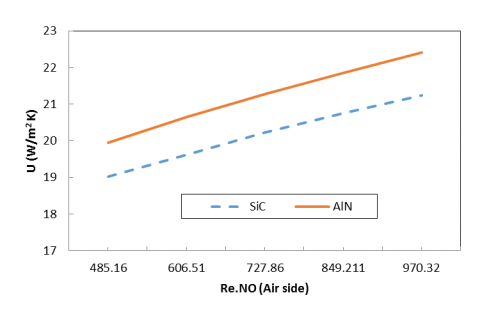Figure 5.Variation of overall heat transfer coefficient of AlNandSiC with Reynolds number

## 6.png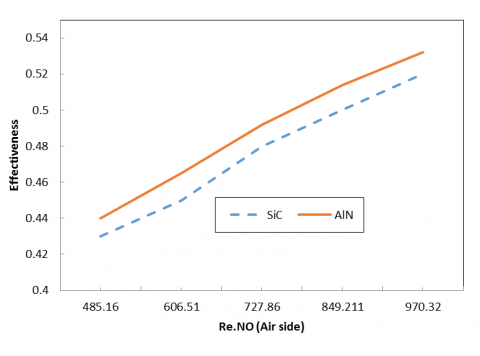Figure 6.Variation of effectiveness of AlNandSiC with Reynolds number

## 7.pngFigure 7.Variation of heat transfer rate of AlN and SiC with Reynoldsnumber

The pressure drop of both the heat exchangers was same at the considered operating conditions since the pressure does not depend greatly on the material property. The calculated pressure drop varied from 13.7-27.47 Pa and the Figure 8 shows the variations of Pressure drop with the Reynolds Number and it increases with the Re.

## 8.png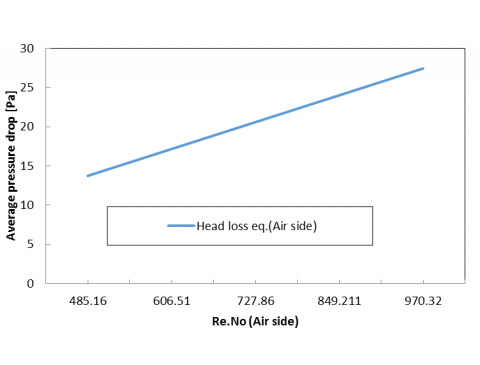Figure 8.Variation of pressure drop with Reynolds number

4. Conclusions

In the presented work, the performance of a ceramic monolith heat exchanger was compared using silicon carbide and aluminium nitride as the heat exchanger material. The theoretical investigations were carried out for the hot exhaust gas, ceramic core, and cold air areas in a ceramic heat exchanger measuring 800-1000°C. The effectiveness and the total heat transfer rates were obtained for silicon carbide material and compared with those calculated for aluminium nitride material. The calculations were carried using a Ɛ-NTU method with various Nusselt number correlations from the literature. It was observed that 5 % rise in overall heat transfer coefficient while 3 % improvement in effectivenessand heat transfer rate was noticed in ceramic heat exchanger using aluminium nitride as the heat exchanger material when compared to silicon carbide material.

Nomenclature
 C Ratio of specific heat Dh Hydraulic diameter h Convective heat transfer coefficient k Thermal conductivity A Heat transfer area Nu Nusselt number NTU Number of transfer unit Pr Prandtl number Re Reynolds number U Overall heat transfer coefficient u Velocity α Aspect ratio ρ Density µ Viscosity Ɛ Effectiveness
References

 Bessette N., Schmitt D.S., Rawson J., Allfather L. (2005). Fuel transformer. Technical progress report semiannual, Acumentircs Practice, Vol. 13, No. 6, pp. 789-803.

 Rokni M., Snden B.A. (1996). Numerical investigation of turbulent force convection in ducts with rectangular and trapezoidal cross-section area by using different turbulence models, Numerical Heat Transfer- Part A- Applications, Vol. 30, pp. 321-346. DOI: 10.1080/ 10407789608913843

 Ranganayakulu C., Seetharamu N.K. (1999). The combined effects of wall longitudinal heat conduction, internal fluid flow non-uniformity and temperature non-uniformity in compact tube-fin heat exchangers: A finite element method, International Journal of Heat and Mass Transfer, Vol. 42, No. 2, pp. 263-273. DOI: 10.1016/S0017-9310(98)00156-2

 Hetsroni G., Mosyak A., Segal Z., Ziskind G. (2002). A uniform temperature hot sink for cooling of electronic device, International Journal of Heat Mass Transfer, Vol. 45, pp. 3275-3286. DOI: 10.1016/S0017-9310(02)00048-0

 Rashidi S., Bovand M., Esfahani J.A. (2015). Heat transfer enhancement and pressure drop penalty in porous solar heat exchangers: A sensitivity analysis, Energy Conversion and Management, Vol. 103, pp. 726-738. DOI: 10.1016/j.enconman.2015.07. 019

 Bouris D., Konstantinidis E., Balabani S., Castiglia D., Bergeles G. (2005). Design of a novel intensified heat exchanger for reduced fouling rates, International Journal of Heat and Mass Transfer, Vol. 48, No. 18, pp. 318-322. DOI: 10.1016/ j.ijheatmasstransfer.2005.03.026

 Park S.K., Choi G.C., Nam H.J., Shin H.D., Park H.S. (2009). A numerical simulation for design of high temperature ceramic heat exchanger, The Korean Society of Combustion, Vol. 14, No. 3, pp. 24-28. DOI: 10.15231/jksc

 Paeng J.G., Yoon Y.H., Kim K.H., Yoon K.S. (2010). Theoretical and numerical analyses of a ceramic monolith heat exchanger, Journal of Mechanical Science and Technology, Vol. 24, No. 7, pp. 1545-1551. DOI: 10.1007/s12206-010-0407-3

 Dizaji H.S., Jafarmadar S., Abbasalizadeh M., Khorasani S. (2015). Experiments on air bubbles injection into a vertical shell and coiled tube heat exchanger; exergy and NTU analysis, Energy Conversion and Management, Vol. 103, pp. 973-980. DOI: 10.1016/j.enconman.2015.07. 044

 Nagarajan V., Chen Y.T., Wang Q.W., Ting M. (2013). Hydraulic and thermal performances of a novel configuration of high temperature ceramic plate-fin heat exchanger, Applied Energy, Vol. 113, pp. 589-602. DOI: 10.1016/j.apenergy.2013. 07.037

 Jian W., Yang H.Z., Wang S.M., Xu S.F., Tuo H.F. (2015). Numerical investigation on baffle configuration improvement of the heat exchanger with helical baffles, Energy Conversion and Management, Vol. 89, pp. 438- 448. DOI: 10.1016/j.enconman.2014.09. 059

 Mahood H.B., Campbell A.N., Thorpe R.B., Sharif A.O. (2015). Heat transfer efficiency and capital cost evaluation of a three-phase direct contact heat exchanger for the utilisation of low-grade energy sources, Energy Conversion and Management, Vol. 106, pp. 101-109. DOI: 10.1016/j.enconman. 2015.09.023

 De Mello P.E.B., Monteiro D.B. (2012). Thermodynamic study of an EFGT (externally fired gas turbine) cycle with one detailed model for the ceramic heat exchanger, Energy, Vol. 45, pp. 497-502. DOI: 10.1016/j.energy.2012.01.003

 Gao Z.L., Liu Y.Q., Gao Z.Q. (2012). Heat extraction characteristic of embedded heat exchanger in honeycomb ceramic packed bed, International Communications in Heat and Mass Transfer, Vol. 39, pp. 1526-1534. DOI: 10.1016/j.icheatmasstransfer. 2012.09.010

 Ma T., Wang Q.W., Zeng M., Chen Y.T., Liu Y., Nagarajan V. (2012). Study on heat transfer and pressure drop performances of ribbed channel in the high temperature heat exchanger, Applied Energy, Vol. 99, pp. 393-401. DOI: 10.1016/ j.apenergy.2012.05.030

 Cadavid Y., Amell A., Cadavid F. (2013). Heat transfer model in recuperative compact heat exchanger type honeycomb: Experimental and numerical analysis, Applied Thermal Engineering, Vol. 57, pp. 50-56. DOI: 10.1016/j.applthermaleng.2013.03.034

 Fend T., Volker W., Miebach R., Smirnova O., Gonsior D., Schollgen D., Rietbrock P. (2011). Experimental investigation of compact silicon carbide heat exchangers for high temperatures, International Journal of Heat and Mass Transfer, Vol. 54, pp. 4175-4181. DOI: 10.1016/j.ijheatmasstransfer.2011.05.028

 Kee R.J., Almand B.B., Blasi J.M., Rosen B.L., Hartmann M., Sullivan N.P., Zhu H.Y., Manerbino A.R., Coors S.M.W.G., Martin J.L. (2011). The design, fabrication, and evaluation of a ceramic counter-flow micro channel heat exchanger, Applied Thermal Engineering, Vol. 31, pp. 2004-2012. DOI: 10.1016/j.applthermaleng.2011.03.009

 Ji Y.P., Ma T., Zeng M., Zhu H.B., Wang Q.W. (2011). Investigation of a novel bayonet tube high temperature heat exchanger with inner and outer fins, International Journal of Hydrogen Energy, Vol. 36, pp. 3757-3768. DOI: 10.1016/j.ijhydene.2010.12.039

 Takeuchia Y., Park C., Noborio K., Yamamoto Y., Konishi S. (2010). Heat transfer in SiC compact heat exchanger, Fusion Engineering and Design, Vol. 85, pp. 1266-1270. DOI: 10.1016/j.fusengdes.2010.03.017

 Delavar M.A., Azimi M. (2012). Using porous material for heat transfer enhancement in heat exchanger: Review, International Journal of Heat and Technology, Vol. 31, No. 2, pp. 93-96. DOI: 10.18280/ijht.300213